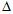# Electrical Engineering - Three-Phase Systems in Power Applications

Exercise : Three-Phase Systems in Power Applications - General Questions
1.
In a three-phase system, the voltages are separated by
45°
90°
120°
180°
Explanation:
No answer description is available. Let's discuss.

2.
In a three-phase system, when the loads are perfectly balanced, the neutral current is
zero
one-third of maximum
two-thirds of maximum
at maximum
Explanation:
No answer description is available. Let's discuss.

3.
In a-connected source driving a-connected load, the
load voltage and line voltage are one-third the source voltage for a given phase
load voltage and line voltage are two-thirds the source voltage for a given phase
load voltage and line voltage cancel for a given phase
load voltage, line voltage, and source phase voltage are all equal for a given phase
Explanation:
No answer description is available. Let's discuss.

4.
In a certain three-wire Y-connected generator, the phase voltages are 2 kV. The magnitudes of the line voltages are
2,000 V
6,000 V
666 V
3,464 V
In a-connected source feeding a Y-connected load,